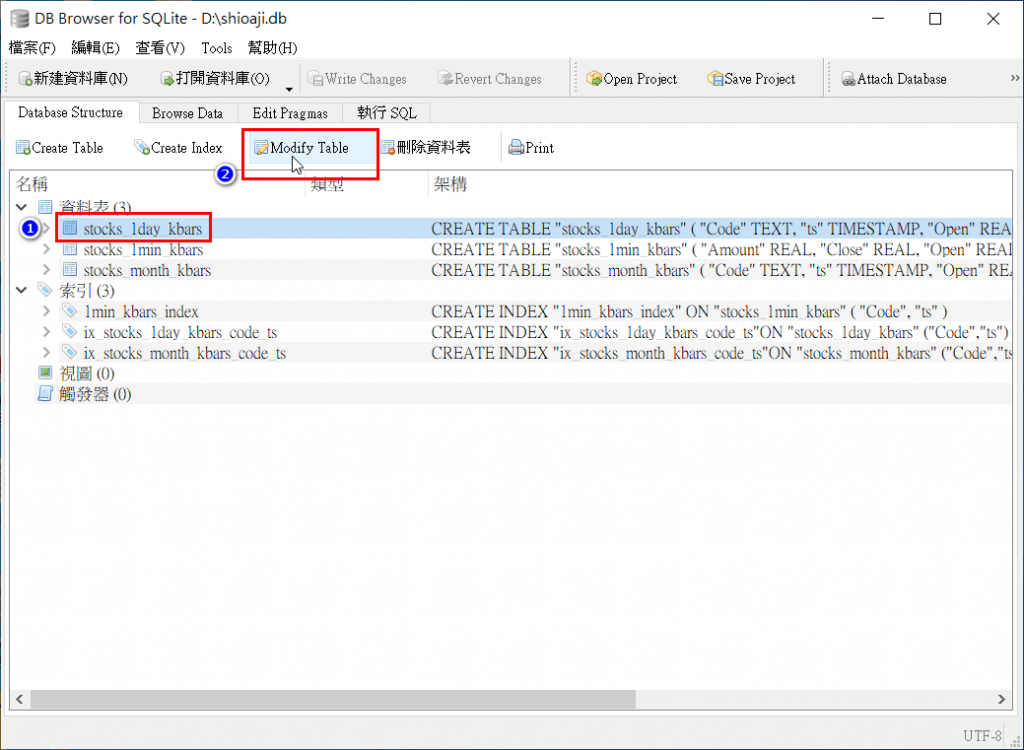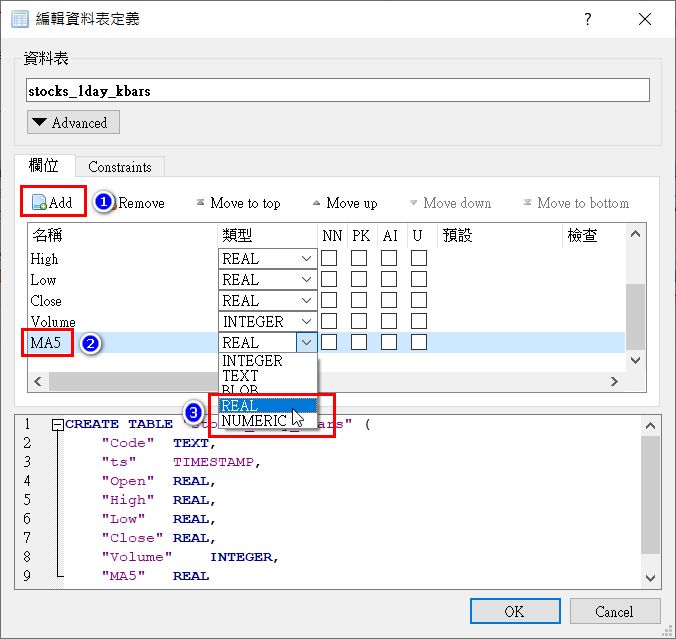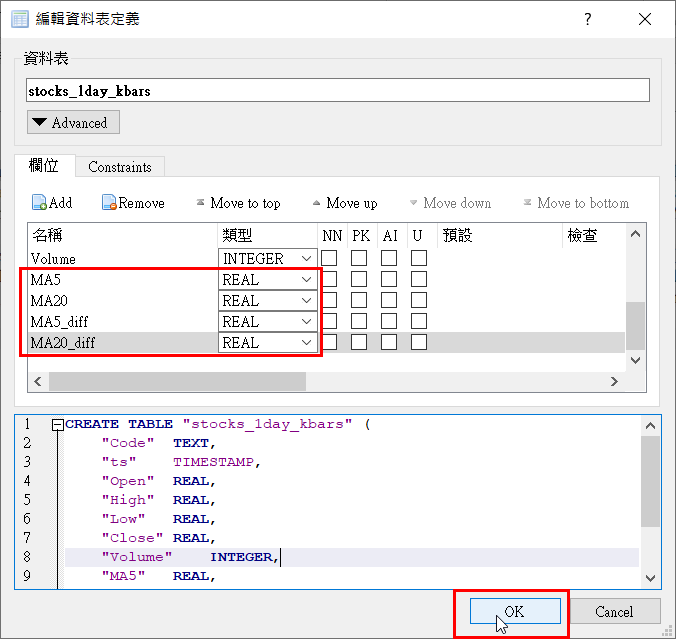DAY 29
0

# 本篇重點

• 在SQLite的Table中，新增欄位
• 透過pandas.DataFrame.rolling計算均線
• 回寫資料至SQLite中

## 在SQLite的Table中，新增欄位## 透過pandas.DataFrame.rolling計算均線

pandas.DataFrame.rolling來計算

``````df['MA5'] = df['Close'].rolling(window=5).mean().round(2) #依照收盤價計算均線，並取至小數點後2位
df['MA20'] = df['Close'].rolling(window=20).mean().round(2)
df['MA5_diff'] = df['MA5'].diff() #計算MA5差異
df['MA20_diff'] = df['MA20'].diff()
``````

## 回寫資料至SQLite中

``````import pandas as pd
import sqlite3
# 更新資料用的SQL語法
UPDATE_SQL = '''UPDATE stocks_1day_kbars
SET MA5 = ?,
MA20 = ?,
MA5_diff = ?,
MA20_diff = ?
WHERE Code = ? AND ts = ?'''
conn = sqlite3.connect('D:/shioaji.db') #建立資料庫連線
# conn = sqlite3.connect('C:/shioaji.db') #建立資料庫連線
cursor = conn.cursor()

# 傳入股票代碼，產生MA均線及差異
def generate_ma_of_day_kbar(stock_code):
print(f'calc MA for stock:{stock_code}')
# 僅取出ts跟Close欄位資料，並將ts欄位設為DataFrame的Index
df = pd.read_sql(f'SELECT ts, Close FROM stocks_1day_kbars WHERE Code = {stock_code}',
conn, index_col='ts')
#依照收盤價計算MA5，並取至小數點後2位
df['MA5'] = df['Close'].rolling(window=5).mean().round(2)
df['MA20'] = df['Close'].rolling(window=20).mean().round(2)
df['MA5_diff'] = df['MA5'].diff() #計算MA5差異
df['MA20_diff'] = df['MA20'].diff()
# 歷遍df中的資料，並update至table中
for index, row in df.iterrows():
cursor.execute(UPDATE_SQL, (row['MA5'], row['MA20'], row['MA5_diff'],
row['MA20_diff'], stock_code, index))
conn.commit()

codes = cursor.execute('SELECT DISTINCT code FROM stocks_1day_kbars').fetchall()
for code in codes:
generate_ma_of_day_kbar(code)

conn.close() #關閉資料庫連線
``````